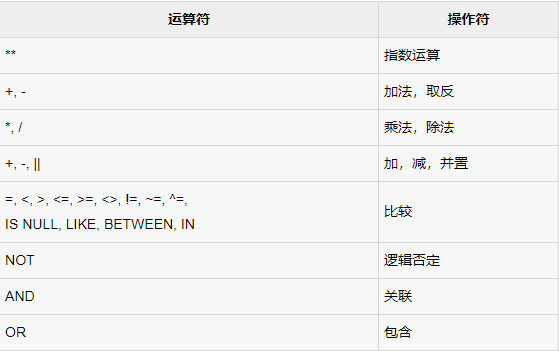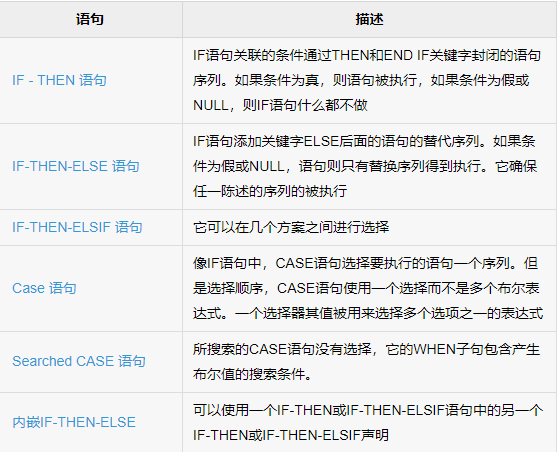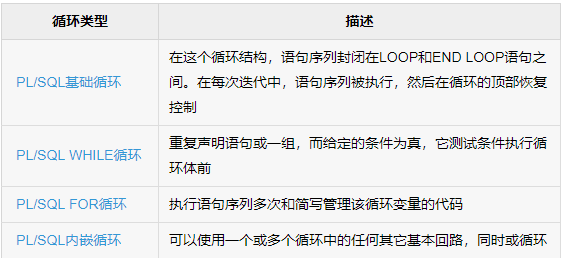# PL/SQL 入门学习（一）

PL/SQL是是由甲骨文公司在90年代初开发，以提高SQL的功能。PL/SQL是嵌入在Oracle数据库中的编程语言之一。PL/SQL是Oracle数据库对SQL语句的扩展。在普通SQL语句的使用上增加了编程语言的特点，所以PL/SQL就是把数据操作和查询语句组织在PL/SQL代码的过程性单元中，通过逻辑判断、循环等操作实现复杂的功能或者计算的程序语言。

declare
msg varchar(20);
begin
msg := 'Hello world ! ';

dbms_output.put_line(msg);

exception
-- 单行注释
/*
* 多行注释 ，这部分是异常处理模块，可有可无。
*/
end；


declare 声明部分 – 所有变量，游标，子程序，和其他元素
begin - end ; – 程序主体，可执行命令

PL/SQL中常见符号解释：
||     字符串连接符
:=    赋值运算符
%    属性索引 使用%type可以使变量获得与字段相同的数据类型，用于不知道给什么类型才合适的变量
=     相等运算符

PL/SQL 变量命名 : 由数字，字母，下划线，美元符号组成。字母开头。

varchar2 : 可变长字符串
int : 整数类型
number (precision,scale) :
1. precision表示数字中的有效位，如果没有指定precision的话，oracle将使用38作为精度；
2. 如果scale大于零，表示数字精度到小数点右边的位数；scale默认设置为0；如果scale小于零，oracle将把该数字取舍到小数点左边的指定位数。

clob: 用于存储字符大块数据在数据库中 大小为 8 - 128 TB
date : 日期和时间

DECLARE
-- Global variables
num1 number := 95;
num2 number := 85;
BEGIN
dbms_output.put_line('Outer Variable num1: ' || num1);
dbms_output.put_line('Outer Variable num2: ' || num2);
DECLARE
-- Local variables
num1 number := 195;
num2 number := 185;
BEGIN
dbms_output.put_line('Inner Variable num1: ' || num1);
dbms_output.put_line('Inner Variable num2: ' || num2);
END;
END;


SQL的SELECT INTO子句给PL/SQL变量赋值

declare
c_id customers.id%type := 1;
c_name customers.name%type;
c_sal customers.salary%type;
begin
dbms_output.put_line('Customer '||c_name||' from '||c_addr||' earns '||c_sal);
end;


PL/SQL 中的常量：

PI constant number := 3.14;

PL/SQL 中的运算符：PL/SQL 中的条件控制语句：DECLARE
a number(3) := 100;
BEGIN
IF ( a = 10 ) THEN
dbms_output.put_line('Value of a is 10' );
ELSIF ( a = 20 ) THEN
dbms_output.put_line('Value of a is 20' );
ELSIF ( a = 30 ) THEN
dbms_output.put_line('Value of a is 30' );
ELSE
dbms_output.put_line('None of the values is matching');
END IF;
dbms_output.put_line('Exact value of a is: '|| a );
END;


CASE 结构：

declare
begin
when 'A' then dbms_output.put_line('A');
when 'B' then dbms_output.put_line('B');
else dbms_output.put_line('NO');
end case;
end;


PL/SQL 中的循环控制语句：DECLARE
x number := 10;
BEGIN
LOOP
dbms_output.put_line(x);
x := x + 10;
IF x > 50 THEN
exit;
END IF;
END LOOP;
-- after exit, control resumes here
dbms_output.put_line('After Exit x is: ' || x);
END;

DECLARE
a number(2) := 10;
BEGIN
WHILE a < 20 LOOP
dbms_output.put_line('value of a: ' || a);
a := a + 1;
END LOOP;
END;

DECLARE
a number(2);
BEGIN
FOR a in 10 .. 20 LOOP   -- 10 .. 20 表示10-20 全包含
dbms_output.put_line('value of a: ' || a);
END LOOP;
END;


UPPER(x) ：x转换为大写字母，并返回该字符串
TRIM([trim_char FROM) x) ： 从左侧和右侧修剪x字符的空格
SUBSTR(x, start [, length]) ： 返回x的一个子开始于由start指定的位置。可选长度为子字符串
CHR(x) ： 返回字符 x 的 ASCII 值
CONCAT(x, y) ： 连接字符串x和y，并返回附加的字符串
INITCAP(x) ： 每个单词的首字母x中转换为大写，并返回该字符串
INSTR(x, find_string [, start] [, occurrence]) ：搜索find_string在x中并返回它出现的位置
INSTRB(x) ： 返回另一个字符串中字符串的位置，但返回以字节为单位的值
LENGTH(x) : 返回x中的字符数
LOWER(x) : 在x转换为小写字母，并返回该字符串
LTRIM(x [, trim_string]) ： 从x的左修剪字符

PS. 以上资料这自于易百教程https://www.yiibai.com/plsql/

06-238万+09-284194
11-221699
04-123万+
03-111775
03-048万+
06-024121
07-241万+
06-154963
09-051241